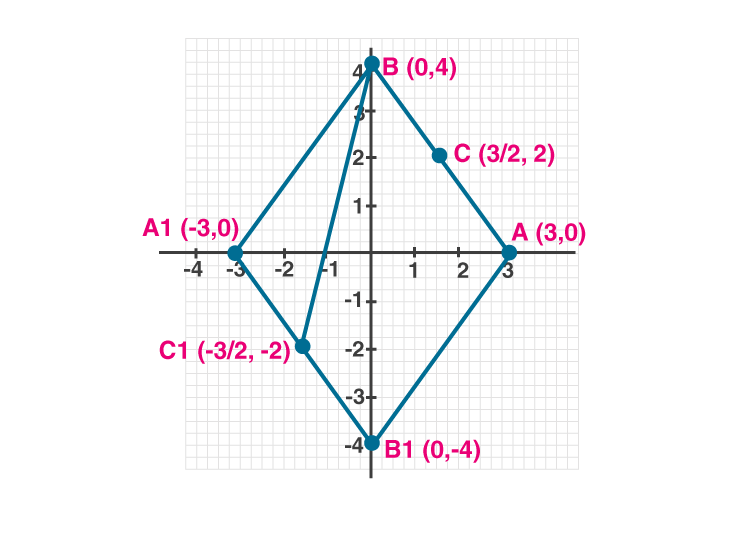Newbie

# Use graph paper for this question. Take 1 cm = 1 unit on both axes. Plot the points A(3, 0) and B(0, 4). (i) Write down the co-ordinates of A1, the reflection of A in the y-axis. (ii) Write down the co-ordinates of B1, the reflection of B in the x-axis. (iii) Assign the special name to the quadrilateral ABA1B1. (iv) If C is the midpoint is AB. Write down the co-ordinates of the point C1, the reflection of C in the origin. (v) Assign the special name to quadrilateral ABC1B1.

• 2

An Important Question of class 10 Based on Section Formula Chapter of M.L Aggarwal for ICSE BOARD.
Here you have point some co-ordinates on the graph as required in question So solve this question.
This is the Question Number 08, Exercise 11 of M.L Aggarwal.

Share

1.(i) Co-ordinates of point A are (3,0).

When you reflect a point across the Y-axis, the y-coordinate remains the same,

but the x-coordinate is transformed into its opposite (its sign is changed)

Hence the reflection of A in the Y axis is (-3,0).

(ii) Co-ordinates of point B are (0,4).

When you reflect a point across the X-axis, the x-coordinate remains the same,

but the y-coordinate is transformed into its opposite (its sign is changed).

Hence the reflection of B in the X-axis is (0,-4)

(iii) The quadrilateral ABA1B1 will be a rhombus.

(iv) Let C be midpoint of AB.

Co-ordinate of C = ((3+0)/2 , (0+4)/2) = (3/2, 2) [Midpoint formula]

In a point reflection in the origin, the image of the point (x,y) is the point (-x,-y).

Hence the reflection of C in the origin is (-3/2, -2)